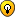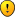## futoshiki anyone?

For fans of all other kinds of logic puzzles

### futoshiki anyone?

I cannibalised my sudoku software to solve futoshiki puzzles. You can download it from here:
http://uk.geocities.com/aidan_001/software.html

Using this software it's possible to create puzzles that the solver can't solve. You create a puzzle for which ther's a cell which has only one valid candidate (the others lead to contradictions) and that candidate solves the puzzle.

I've posted such a puzzle on the link above. I'd be interested to know what techniques can be used to solve it. (I'm guessing people are going to suggest forcing chains, but hoping there are some more elegant techniques that I'm not aware of).

As my page is a free geocities page, I haven't included a direct link to the picture in this thread, but if anyone has some spare bandwith and wants to copy the picture to their page to provide a picture in this thread, please feel free.
Last edited by ab on Sun Jun 17, 2007 8:29 am, edited 1 time in total.
ab

Posts: 451
Joined: 06 September 2005

I guess your program has at least one other technique to make use of the inequality signs.

So far I see for example the following basic technique is not used:

Let R1C1<R1C2>R1C3>R1C4

from this follows R1C2 has 3 smaller values in his row => R1C3 > 3

The same works in the other direction

R1C1>R1C2<R1C3<R1C4 => R1C2 < 3 (in a 5x5 grid)

we could call this "number of greater cells" (in a line) or number of smaller cells" (in a line). This works also in boxes with greater/less sudoku.

In greater futoshiki you can also expand this technique not only using the given signsbut also using signs that can be concluded by the former steps. If for example cell R1C1={456} and R1C9={789} so you can conclude R1C1<R1C9. This works still with R1C1={4567} and R1C9={789}.

And you can refine these techniques using the possible values of the greater or less cells.

I know these technques doesn't help in the example on your page but in many other cases (at least with bigger grids).

You can use these techniques in this futoshiki:Pyrrhon

Posts: 240
Joined: 26 April 2006

Pyrrhon wrote:I guess your program has at least one other technique to make use of the inequality signs.

So far I see for example the following basic technique is not used:

Let R1C1<R1C2>R1C3>R1C4

from this follows R1C2 has 3 smaller values in his row => R1C3 > 3

The same works in the other direction

R1C1>R1C2<R1C3<R1C4 => R1C2 < 3 (in a 5x5 grid)

These are the standard eliminations made by the program, I haven't included it as a separate technique. If you create an empty grid and set up your example, you'll see that the only remaining candidates in r1c2 are 3, 4 or 5.

Thanks for the feedbackab

Posts: 451
Joined: 06 September 2005

You doesn't meet my point. Yes, the program gets the 3 possibilities {345}. But we have more information. It can't be 3, it must be greater then 3, because there are 3 smaller digits in this row (R1C1,R1C3,R1C4). So R1C2 should only have the candidates 4 and 5.

This works still in the following situation: R2C2>R1C2, R1C1<R1C2>R1C3
Here R2C2 again has only the possibilities 4 and 5.

Pyrrhon
Pyrrhon

Posts: 240
Joined: 26 April 2006

ahOK thanksI'm new to futoshiki, I basically just thought what ideas from sudoku will work for futoshiki. I'll have to add that to my armoury.
ab

Posts: 451
Joined: 06 September 2005

For the puzzle your solver can't solve:I found the trickiest step to be (spoiler warning):

The trickiest step

One of the 2 yellow cells must be 4, so at least one of the 2 green cells must be 5, therefore the red cells cannot be 5

And here is my complete walkthrough... See if you can pick up some techniques for your program...r4c2345 all have larger neighbours => r4c1=5
r2c1245 all have larger neighbours => r2c3=5
r2c2<r1c2<r1c3 => r2c2<4, r1c2<5
r2c1<r1c1<r4c1=5 => r2c1<4, r1c1<5
r2c5<r2c4<r2c3=5 => r2c5<4, r2c4<5
=> r2c4=4 (hidden single on r2)
r4c3,r5c4<r4c4<r3c4 & r2c4=4 => r4c3<3, r5c4<3, r4c4<4
=> One of r4c25 must be 4, with r4c2<r3c2 & r4c5<r5c5
=> At least one of r3c2 & r5c5 must be 5
=> r3c5<5, r5c2<5
r1c2<5, r2c2<5, r5c2<5 & r4c1=5 => r3c2=5
r5c2<5, r5c4<3 & r4c1=r2c3=5 => r5c5=5
r4c3,r5c4<r4c4<r3c4 & r2c4=4, r3c2=5
=> r4c3=r5c4=1, r4c4=2, r3c4=3 => r1c4=5
r1c123 all have smaller neighbours => r1c5=1
r2c2=1 (hidden single on c2)
r2c5<r3c5 & r4c4=2 => r2c5=2 => r2c1=3 => r1c1=4
r1c2<r1c3 => r1c2=2, r1c3=3
r3c4=3 => r3c5=4 => r4c5=3 => r4c2=4 => r5c2=3
r4c3=1 => r3c1=1, r3c3=2 => r5c1=2, r5c3=4udosuk

Posts: 2698
Joined: 17 July 2005

Your trickiest step is the only step that ab's program missed to solve the puzzle. Well observed.

Pyrrhon
Pyrrhon

Posts: 240
Joined: 26 April 2006

thanks for that udosuk, exactly what I was looking forIt also solves another puzzle I had found, or at least a variation of your trick does.
ab

Posts: 451
Joined: 06 September 2005

I've long since updated the software to cope with udosuk's technique, as well as some extensions of itAlso just today I uploaded a version with nice loops on multiple candidates, which helps it solve some more puzzles. Not sure why it took me so long to think of that!
ab

Posts: 451
Joined: 06 September 2005

This solver has evolved again to include a technique that combines colours and inequalities. I've also uploaded a pdf file explaining some futoshiki solving techniques.

All of which can be found here:
http://uk.geocities.com/aidan_001
ab

Posts: 451
Joined: 06 September 2005

Return to Other logic puzzles